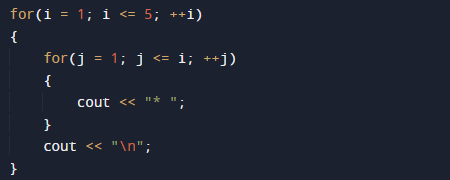# C++ Program to Print Triangle of Star

In this tutorial you will learn about the C++ Program to Print Triangle of Star and its application with practical example.

In this tutorial, we will learn to create a c++ program that will create a Triangle of Stars using c++ programming.

## Prerequisites

Before starting with this tutorial we assume that you are best aware of the following C++ programming topics.

• if-else Statements.
• Looping statement.
• Basic input/output.
• Operators.

## Triangle of Star

To print Triangle Star patterns in C++ , we will use two nested loops. The outermost loop is responsible for the number of rows in star (*) pyramid triangle,whereas inner one  loop will print the required number of stars * in each row.

## C++ Program to Creating a Triangle of Star.

In this program we will print Triangle of Star using nested  for loop. We would first declared and initialized the required variables. Then we will create the Triangle of Star using below program.

## OutputIn the above program, we have first declared and initialized a set variables required in the program.

• i & j= for  iteration.

In above program  first we declare variables i and j, here we use 2 for loops.The outer loop will run for number of rows or pyramid and the inner loop print the number of stars to be displayed in each row.This is displayed using the following code.And the final output of our program is like that.In this tutorial we have learn about the C++ Program to Print Triangle of Star and its application with practical example. I hope you will like this tutorial.